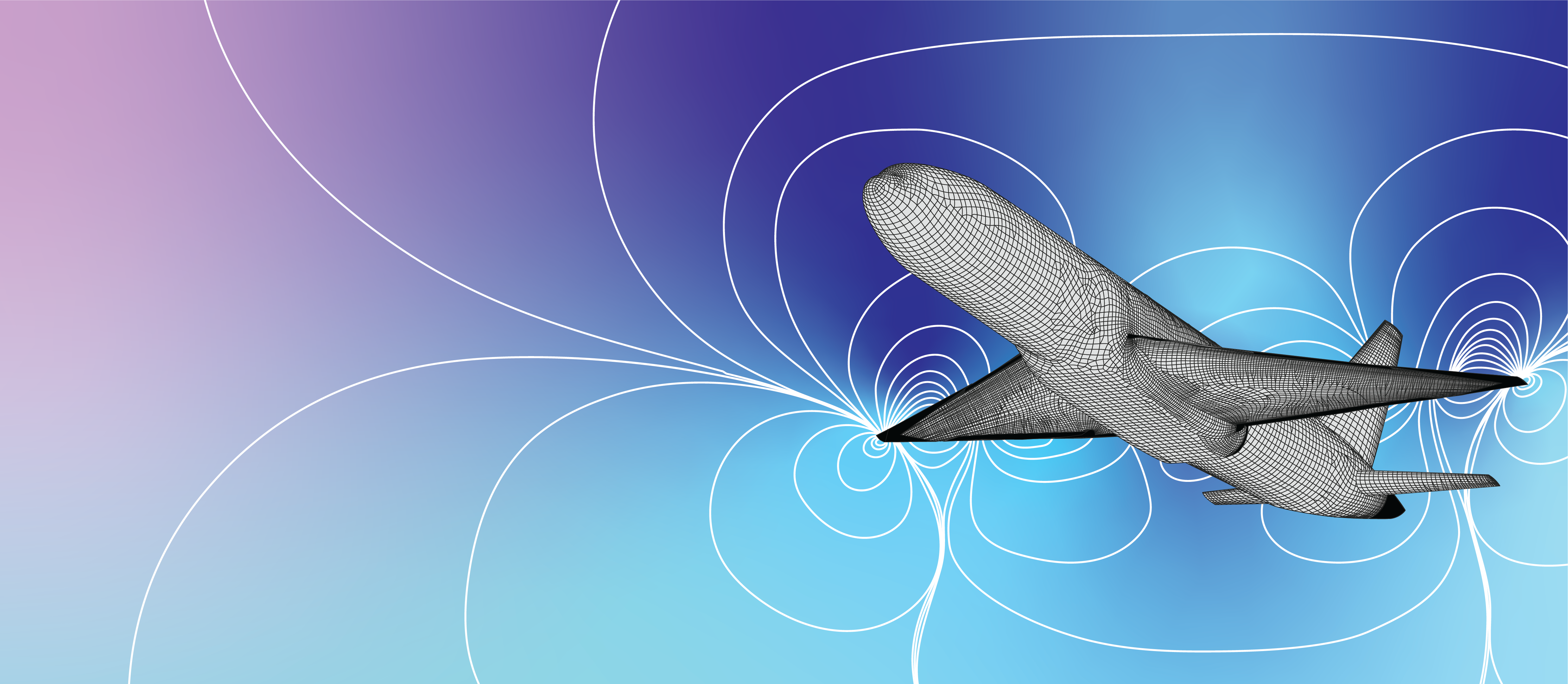## Shock developments

### When it comes to aeroengineering, we don't wing it

Designs by Nicolette Puksar

May 23, 2023How is an airplane in motion like water gushing through a pipe? Though seemingly unrelated, both situations are examples of fluid flows, meaning they involve the motion of liquids and gases. These phenomena, like the turbulence on an airplane in flight, have analogues to rapidly flowing water. In fact, both situations, and other fluid flows in general, can be described by the general framework of equations of fluid motion. These equations describe the rate of change of the fluid’s speed and its flow direction at a specific point in terms of variables such as regional pressure and density.

The aircraft in motion is a prime example that UC Berkeley graduate student Lewis Pan and Dr. Per-Olof Persson, both from the Department of Mathematics, use to illustrate the study of the equations of fluid motion. In this example, an interesting phenomenon becomes apparent at high speeds. An aircraft traveling through air results in disturbances in the air: these disturbances, which are analogous to the ripples produced when a stone is thrown into a lake, are caused by molecules colliding with each other and traveling at the speed of sound. However, if the aircraft is moving faster than the speed of sound, these disturbances are not propagated to new locations before the aircraft reaches them. “The result is the formation of regions with drastic fluid flow variations, called shocks”, notes Pan, adding that “near a shock, physical quantities like air pressure may change abruptly”.

Understanding shocks has practical importance in various fields, such as designing supersonic jets. In the specific case of designing supersonic jets, one must ensure that the pressure differences resulting from the shocks do not threaten the structural integrity of the aircraft. Consequently, carefully analyzing the equations of fluid motion is important. Unfortunately, these equations are complicated; in all but the simplest cases, it is impossible for a human to solve them on paper.

Luckily, there is an alternative strategy—attempting to solve the equations using a computer. This approach involves discretization, wherein the region of fluid flow space is represented by many, finite points, which are arranged in a possibly irregular grid, called a mesh. This translation is like converting an image into pixel art, where the goal is to reproduce a closely, resembling image using a grid of colored points.

In this manner, the complex problem of solving the equations of motion is reduced to only needing to solve the equations of motion at each point on the mesh. This method trades a small amount of accuracy for a substantial gain in simplicity and thus produces an approximate solution to the equations of motion.

As in the toy example of an aircraft in supersonic motion, the objective is to produce a mesh representing the surface of the aircraft and use it to produce an approximate solution to the equations of motion of the surrounding air. However, researchers working on this problem encounter complications. The main challenge is to ensure that the locations of shocks are incorporated into the mesh to prevent inaccurate solutions, since variables like air pressure change drastically near a shock. Additionally, designing a mesh in this situation is itself a difficult task, as the surface of an aircraft is a complicated geometric shape. Therefore, careful design of the mesh is necessary to minimize any errors.

For many problems in numerical analysis, choosing an appropriate mesh is an important question that guides research, including that of Pan and Persson. “Our idea is to calculate the locations of the shock beforehand to make sure that the mesh aligns with the shock”, Pan explains, noting that this foresight also incorporates the drastic variation produced by the shock into the mesh. Implementing this idea is itself an intricate process because locating the shocks is a non-trivial problem. Since shocks are small regions of abrupt change, the broad idea is to scan the entire region for points where the variation is as large as possible.

The research done by the UC Berkeley team hopes to have a variety of applications. The technical analysis behind choosing the mesh appropriately represents progress in numerical analysis. “As of now, nobody has figured out how to fully deal with shocks,” Pan remarks. In addition to a more robust understanding of shock waves on supersonic aircraft, fluid flow modeling has applications in other fields—in seismology, for instance, progress in understanding shocks would lead to significant improvement in modeling earthquakes.

## Recent Articles

•••## Most Popular

••Notice something wrong?